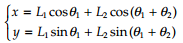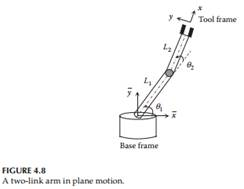### Create an Account

Home / Questions / A planar two-link robot arm is shown in Figure 4.8 The coordinate system xy is the tool fr...

# A planar two-link robot arm is shown in Figure 4.8 The coordinate system xy is the tool frame and is attached to the end-effector The coordinates of the end-effector relative to the base frame are

A planar, two-link robot arm is shown in Figure 4.8. The coordinate system xy is the tool frame and is attached to the end-effector. The coordinates of the end-effector relative to the base frame are expressed asSuppose the lengths, in consistent physical units, of the two links are L1 = 1 and L2 = 2, and that x = 2.5, y = 1.4. Find the joint angles θ1 and θ2 (in radians) using Newton’s method with an initial estimate of (0.8, 0.9), tolerance ε = 10−4, and maximum number of iterations set to 10.Jul 22 2020 View more View LessSubscribe To Get Solution